## Logarithmic Binomial Theorem

For all integersand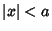,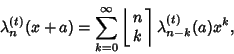whereis the Harmonic Logarithm andis a Roman Coefficient. For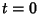, the logarithmic binomial theorem reduces to the classical Binomial Theorem for Positive, sincefor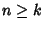,for, andwhen.

Similarly, taking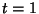and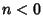gives the Negative Binomial Series. Roman (1992) gives expressions obtained for the caseandwhich are not obtainable from the Binomial Theorem.

Roman, S. The Logarithmic Binomial Formula.'' Amer. Math. Monthly 99, 641-648, 1992.